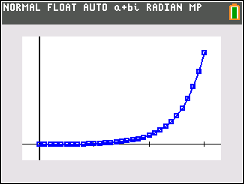## Monday, March 13, 2017

### TI-84 Plus (and General Graphing): Semi Log Plots (LINLOG)

TI-84 Plus (and General Graphing):  Semi Log Plots (LINLOG)

Introduction

Many applications, such as the Bode Plot, are plotted as a semi-log plot.  In particular, a lin log plot is used.   In a lin log plot, the x-axis is on a logarithmic scale, while the y-axis is a linear scale.

Graphing calculators do not have a lin log plot mode (at least not that I know of).  However, we can simulate lin log plots.

How to Simulate Lin Log Plots

We are going to enter Function plotting mode (y(x)) as usual.  The trick here is to substitute every x with 10^x or ALOG(x).   For example:

y(x) = 5*x – 2  becomes
y(x) = 5 * 10^x – 2  or y(x) = 5 * ALOG(x) – 2

In essence, we think of the x axis not as {0, 1, 2, 3, …} but {10^0, 10^1, 10^2, 10^3, …. }.

Caution:  With certain functions, the range can grow quickly.  Be careful on how you set the axis.

Reference:  10^0 = 1, 10^1 = 10, 10^2 = 100, 10^3 = 1,000

The TI-84 Plus program LINLOG uses a list plot of a function and sets the axis to the appropriate settings.

TI-84 Plus Program LINLOG

PlotsOff
FnOff
Disp "LOGARITHMIC X AXIS"
Disp "10^{0,1,2,...,N}"
Disp "USE QUOTES"
Input "Y = ",Y
Input "TO 10^N: ",N
{0}→L1
10^(0)→X
{Y1}→L2
For(I,0,N,0.1)
10^(I)→X
augment(L1,{I})→L1
augment(L2,{Y1})→L2
End
Plot1(xyLine,L1,L2)
ZoomStat

Be sure to use quotations when entering the function.  Remember that X in this case represents 10^x.    Below are example plots.y = x / 2

Try it, I hope you find this helpful.  Thank you for your support and comments.  See you next time,

Eddie

This blog is property of Edward Shore, 2017

#### 1 comment:

1.Thank you very much, it was of great help

### TI-84 Plus and Casio fx-CG50: Which Die Wins?

TI-84 Plus and Casio fx-CG50: Which Die Wins? Introduction:  Which Die Wins?   The program DICEODDS compares a pair of dice against eac...